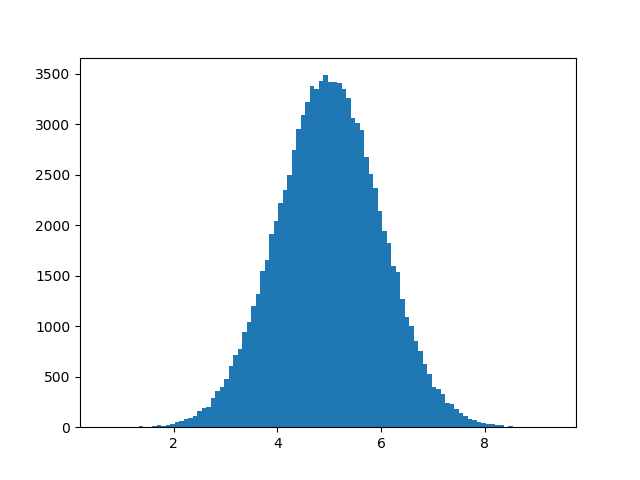# 机器学习 - 正态数据分布

## 正态数据分布（Normal Data Distribution）

### 实例

```import numpy
import matplotlib.pyplot as plt

x = numpy.random.normal(5.0, 1.0, 100000)

plt.hist(x, 100)
plt.show()
```

### 结果：